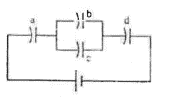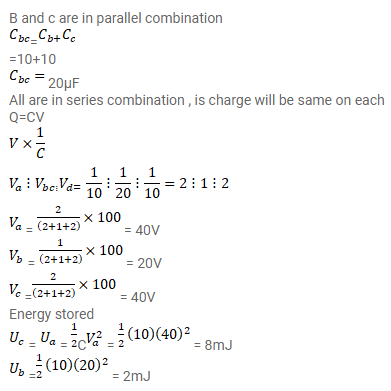# Each capacitor in figure has a capacitance ofQuestion:

Each capacitor in figure has a capacitance of $10 \mu \mathrm{F}$. The emf of the battery is $100 \mathrm{~V}$. Find the energy stored in each of the four capacitors.Solution: